Courses

# Discrete Simulation Models - 2 Civil Engineering (CE) Notes | EduRev

## Civil Engineering (CE) : Discrete Simulation Models - 2 Civil Engineering (CE) Notes | EduRev

The document Discrete Simulation Models - 2 Civil Engineering (CE) Notes | EduRev is a part of Civil Engineering (CE) category.
All you need of Civil Engineering (CE) at this link: Civil Engineering (CE)

Stochastic Cellular Automata (STCA)

We shall now discuss stochastic TCA models (i.e., these are probabilistic CAs) that allow for the spontaneous emergence of phantom jams. All these models explicitly incorporate a stochastic term in their equations, in order to accomplish this kind of real-life behaviour.

In 1992, Nagel and Schreckenberg proposed. A TCA model that was able to reproduce several characteristics of real-life traffic flows, e.g., the spontaneous emergence of traffic jams. Their model is called the NaSch TCA, but is more commonly known as the stochastic traffic cellular automaton (STCA). It explicitly includes a stochastic noise term in one of its rules, which we present in the same fashion as those of the previously discussed deterministic TCA models. The space is divided into cells (cell may contain vehicle or can be empty). The length of a cell is the minimum space headway available between vehicles in times of jam, and numerically it is reciprocal of jam density and is set to 7.5 m( Kj=133veh/km).

The STCA then comprises the following three rules (note that in Nagel and Schreckenberg’s original formulation, they decoupled acceleration and braking, resulting in four rules). Here we shall discuss about the NaSch model based upon its rules. There are four rules, mainly rules for acceleration, rules of deceleration, rules for randomization and lastly the vehicle updation step.

Step 1: Rule for acceleration

if(v< vmax), then vn −→ min(v + 1, vmax)                                                                    (20.5)

This step reflects the general tendency of the drivers to drive as fast as possible without crossing the maximum speed limit. If the present speed is smaller than the desired maximum speed, the vehicle is accelerated. The desired speed vmax can be assumed to be distributed by a statistical distribution function where the values of vmax are only allowed to be 1, 2,..., 5 cell/∆t.

Step 2: Rule for deceleration

if(d≤ vn), then vn−→ min(vn, dn − 1)                                                                           (20.6)

This step ensures that the driver doest collide with any vehicle ahead of him so that deceleration is applied to those vehicles which may collide. If the present speed is larger than the gap in the front, set v = gap. This rule avoids rear end collisions between vehicles. Note that here a very unrealistic braking rule allowing for arbitrarily large decelerations is involved. This rule forces minimum time headway of ∆t s.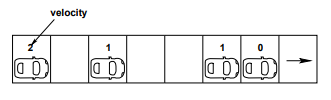Step 3: Rule for randomization if(v > 0), then v−→ max(v − 1, 0)                              (20.7)

This step of randomization takes into account the different behavioral patterns of the individual drivers, especially, overreaction while slowing down and nondeterministic acceleration where overreaction while slowing down will be mostly responsible for the formation of traffic jams.

This rule introduces a random element into the model. This randomness models the uncertainties of driver behavior, such as acceleration noise, inability to hold a fixed distance to the vehicle ahead. Fluctuations in maximal speed, and assign different acceleration values to different vehicles. If present velocity of a vehicle is greater than zero then the velocity of the vehicle reduces by a single unit with a probability Pbrake. This rule has no theoretical background and is introduced quite heuristically.

Step 4: Vehicle updation

x −→ x + v                                                                                                                   (20.8)

After the above three steps the position of vehicles are updated according to their respective velocities. Even changing the precise order of the steps of the update rules stated above would change the properties of the model.

Numerical Example

Assume a single lane stretch road divided into 8 cells and vehicles are present in the first, third, sixth, seventh cells with 2, 1, 1, 0 as their velocities respectively. Apply the rules of cellular automata.

Solution Apply the CA rules (equation. 20.5 - 20.8) in a sequential way as per the requirements of acceleration, deceleration, randomization and vehicle updation. The rules are applied step wise as shown below.

Solution Step 1: Acceleration stage (according to equation. 20.5)

if(vn < vmax, then vn −→ min(vn + 1, vmax)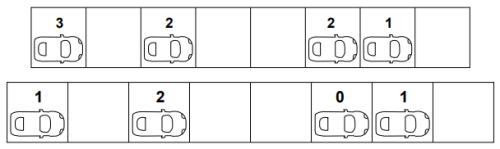Here the velocity of the present vehicle is 2 where the maximum velocity is 5 so the vehicle gets accelerated and acquires the new velocity based upon the min of the (present velocity (2) +1, or the maximum velocity).

• First vehicle: (2 < 5) so min(2 + 1, 5) = 3. Similarly applying the same rule for the rest of the vehicles the velocities acquired are as follows.

• Second vehicle: (1 < 5) so min(1 + 1, 5) = 2

• Third vehicle: (1 < 5) so min(1 + 1, 5) = 2

• Fourth vehicle: (0 < 5) so min(0 + 1, 5) = 1

Step 2: Deceleration stage ( according to equation. 20.6)

if(dn ≤ vn), then vn −→ min(vn, dn − 1)

In this step the vehicle decelerates if it doest find enough gap ahead of it in its lane. The new velocity of the first vehicle is 3 where as the gap ahead of it 2 so it needs to decelerate by an amount of gap minus one i.e., (2-1)=1.

• First vehicle : ( 2 3) , min ( 2-1, 3) = 1 Similarly applying it to remaining vehicles the updated velocities are obtained and are shown below.

• Second vehicle : (3 > 2), no deceleration.

• Third vehicle : (1 < 2), (1 − 1, 2) =0

• Fourth vehicle : (2 > 1), no deceleration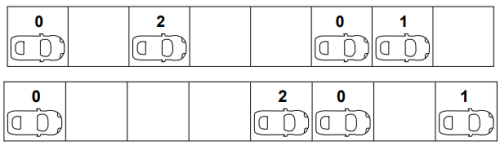Step 3: Randomization stage ( according to equation. 20.7)

if(vn > 0), then vn−→ max(vn − 1, 0)

This step is generally a randomly applied rule for a particular number of vehicles in a set of total n vehicles. This number depends upon the probability ratio p that the user defines. But in our case as we are working with a limited number of vehicles so we cannot use the probability function so for the simplicity of the rule we shall apply this rule ( additional deceleration) to all the those vehicles which undergo deceleration stage. So in our case the first vehicle undergoes randomization stage and acquires a new velocity of 0.

• First vehicle : (1 > 0), max(1 − 1, 0) = 0

Step 4: Vehicle updation:

( according to equation. 20.8)

xn −→ xn + vn

The velocity of the first vehicle after undergoing the three rules has been reduced from two to zero, so the position of the vehicle is not changed in the next time step.

• First vehicle : Xn = 1 + 0 = 1 Similarly applying the rule to the rest of the vehicles in the same way will obtained the following results as below.

• Second vehicle :Xn = 3 + 2 = 5

• Third vehicle : Xn = 6 + 0 = 6

• Fourth vehicle :Xn = 7 + 1 = 8

The figure below gives the reader a clear overview if all the four stages at a glance.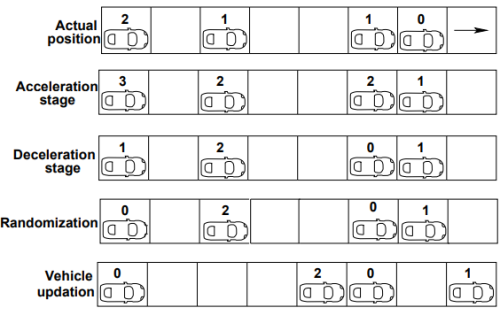Limitations

• Every model has some limitations and as such this cellular automata for single lane traffic has also some limitations which are stated below.

• A single lane model doesn’t suit the realistic traffic where it has vehicle types of different velocities. So here in single lane model if such vehicles are entertained the result is the platooning effect and the average velocity of the stream becomes the velocity of slow moving velocity.

• So, two lane models are introduced to meet the requirement and four more additional rules are included for the exchange of vehicles between the lanes.

Lane changing

The concept of lane changing came into picture with the disadvantage of the single lane model of unexplained realistic traffic conditions. The reason behind this disadvantage is that a realistic traffic is usually composed of vehicle types of different desired velocities.

The presence of such vehicles will result in platooning effect. The generic two lane model is the combination of two parallel single lane models with periodic boundary conditions with some additional rules as stated in the below sections. The update step is split into two sub-steps. In first sub-step the exchange of vehicles in the two lanes, take place according to the new rule set. Vehicles are moved only sideways. They do not advance in one go. However, in reality this does not happen and this is step is also not seen. This step has a meaning when it is coordinated with the second step. In the second step, the independent single-lane updates on both lanes according to the single lane update rules.

STCA models of two lane traffic:

Nagatani has formulated an oversimplified model for two-lane traffic. There are some assumptions in this model, one of which is that the maximum allowable speed of each vehicle is identical. Therefore, the model turns out to be a homogeneous type model and hence cannot explain heterogeneous traffic consisting of different types of vehicles. Some of the notations, which will be used to indicate the gaps in the lanes, are

• ∆Xf p (n) = gaps in front of nth vehicle in its present lane.

• ∆Xf o (n) = gaps in front of nth vehicle in other lane.

• ∆Xb o (n) =gap in the other lane behind the site.

All lane-changing rules consist of two parts: Trigger criterion (“Do I want to change the lane”) and Safety criterion (“Is it safe if I change the lane”). Once if both the criteria are fulfilled, the vehicle will change the lane.

In a two lane model proposed by Rickert, a vehicle changes its lane with a probability p, provided there is not enough gap in the current lane in front of the vehicle, if the gap in the front of the vehicle in the target lane is adequate, if it is possible without collision and finally when the lane changing activity doesn’t block someone else’s way. The above sentences are formulated in form of rules from equations. 20.9 to 20.11

• The vehicle does not find enough gap in its current lane ahead of it.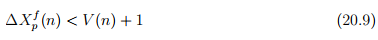• The gap in the target lane ahead of it is adequate.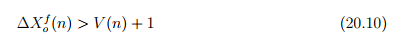• No collision takes place (i.e.,The cell where the vehicle is intending to change should be empty)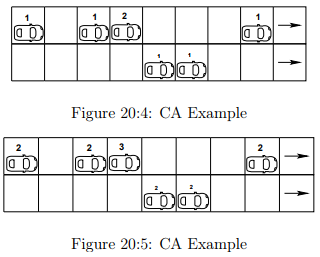• It should not block some others way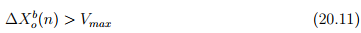Rules 9 and 10 are called the trigger criterion and the rule 11 is called the safety criterion. These rules are applicable for both left to right and right to left lane changes and it changes the lane with probability.

Offer running on EduRev: Apply code STAYHOME200 to get INR 200 off on our premium plan EduRev Infinity!

,

,

,

,

,

,

,

,

,

,

,

,

,

,

,

,

,

,

,

,

,

;